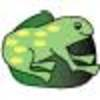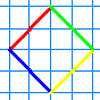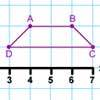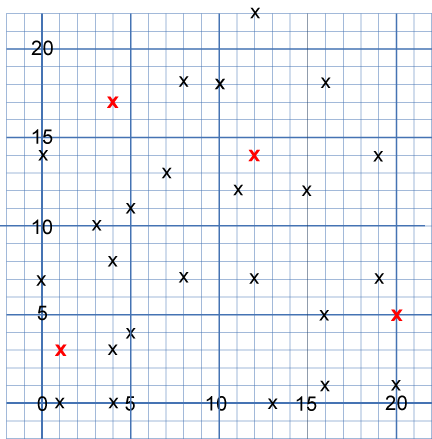#### You may also like### The Lily Pond

Freddie Frog visits as many of the leaves as he can on the way to see Sammy Snail but only visits each lily leaf once. Which is the best way for him to go?### A Cartesian Puzzle

Find the missing coordinates which will form these eight quadrilaterals. These coordinates themselves will then form a shape with rotational and line symmetry.### Transformation Tease

What are the coordinates of this shape after it has been transformed in the ways described? Compare these with the original coordinates. What do you notice about the numbers?

# Eight Hidden Squares

##### Age 7 to 14Challenge Level

On the graph below there are $28$ marked points.These points all mark the vertices (corners) of eight hidden squares.
Each of the $4$ red points is a vertex shared by two squares.
The other $24$ points are each a vertex of just one square.
All of the squares share just one vertex with another square.
All the squares are different sizes.
There are no marked points on the sides of any square, only at the vertices.

Can you find the eight hidden squares?